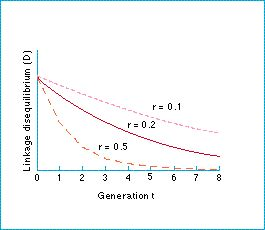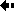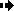## Multi-locus population genetics - How does the amount of linkage disequilibrium change?A balance between recombination and selection

In the absence of selection and in an infinite random mating population, the amount of linkage disequilibrium undergoes exponential decay at a rate equal to the recombination rate between the two loci, as shown by the figure.

In other words, the difference between the actual frequency of a haplotype and its random proportion decreases each generation by a factor equal to the recombination rate between the loci.

Over time, any non-random gene associations will disappear; recombination will destroy the association. The higher the rate of recombination, the more rapid the destruction. The highest possible value of r is 1/2, which is true when the two loci are on different chromosomes. Gene associations persist longer for tightly linked loci on the same chromosome.

We can now see why random gene frequencies are called an 'equilibrium'. In the absence of selection, the action of recombination over time will drive the haplotypes to these frequencies and then keep them there.

Figure: recombination between the loci breaks down the linkage disequilibrium, which decays at an exponential rate equal to the recombination rate between the loci.Previous Next